# Probability distribution facts for kids

Kids Encyclopedia Facts

Probability distribution is a term from mathematics. Suppose there are many events with random outcomes. A probability distribution is the theoretical counterpart to the frequency distribution. A frequency distribution simply shows how many times a certain event occurred. A probability distribution says how many times it should have occurred in the long run (that is, its probability). The probability distribution of a random variable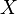$X$ is often written as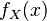$f_X(x)$ (or simply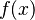$f(x)$). Such a distribution can either be discrete, taking a discrete (or countable) amount of values, or continuous, taking an uncountable amount of values (as from a continuous interval).

As an example, the probability distribution for a single roll of a normal 6-sided dice can be presented by:

 Result Probability of result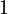$1$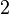$2$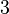$3$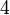$4$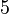$5$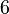$6$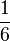$\frac {1}{6}$$\frac {1}{6}$$\frac {1}{6}$$\frac {1}{6}$$\frac {1}{6}$$\frac {1}{6}$

where result is the outcome of the dice roll, and the probability shows the chances of that result occurring. If we roll a dice 60 times, then in the long run, we should expect to have each side appear 10 times on average.

There are different probability distributions. Each of them has its use, its benefits and its drawbacks. Some common probability distributions include:

## Images for kids

it:Variabile casuale#Distribuzione di probabilitàProbability distribution Facts for Kids. Kiddle Encyclopedia.